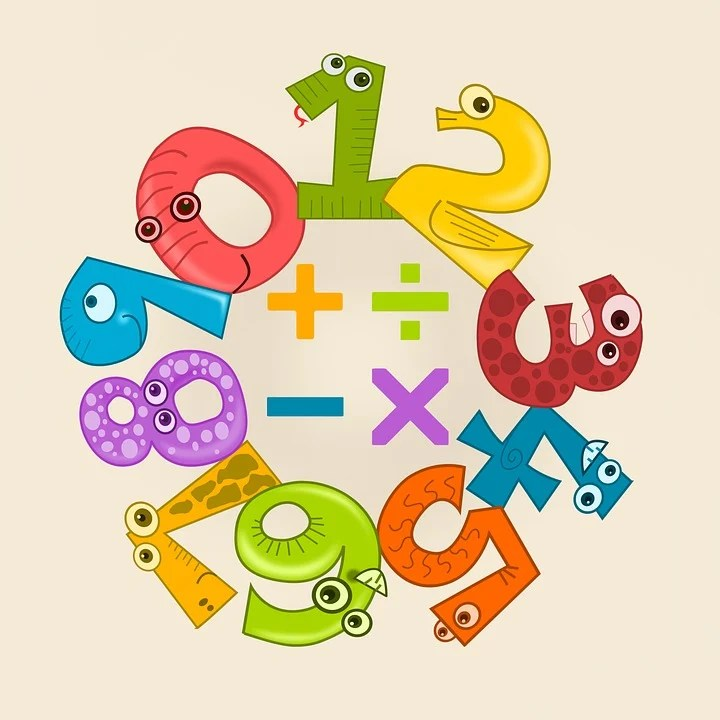## Multiplication – multiply by 2, 5 and 10.

Multiply by 2 | Learn Multiplication Song

Multiply by 5 – Learn Multiplication

Multiply by 10 | Learn Multiplication

More funky songs…

Two Times Table Song (Cover of Rude by MAGIC!) with Classroom Instruments

Five Times Table Song (We Can’t Stop by Miley Cyrus) Using iPads Only

Ten Times Table Song! (Cover of Look What You Made Me Do by Taylor Swift)

Multiplying by 2

Multiplying by 5

Multiplying by 10

## You are now ready to complete these fun multiplication worksheets.Today Year 2 are learning Multiplication – multiply by 2, 5 and 10. They will complete a FREE printable maths worksheet and practice this skill.

Click here to see the previous lesson – Year 2 Week 2 Day 2 Maths Lessons

Click here to see the next lesson – Year 2 Week 2 Day 4 Maths Lessons